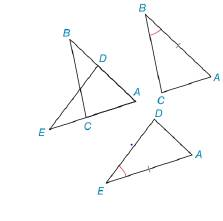Chapter 3.1, Problem 19E### Elementary Geometry for College St...

6th Edition
Daniel C. Alexander + 1 other
ISBN: 9781285195698

#### Solutions

Chapter
Section### Elementary Geometry for College St...

6th Edition
Daniel C. Alexander + 1 other
ISBN: 9781285195698
Textbook Problem
1 views

# In Exercises 19 and 20, the triangles to be proved congruent have been redrawn separately. Congruent parts are marked.a) Name an additional pair of parts that are congruent by using the reason Identity.b) Considering the congruent parts, state the reason why the triangles must be congruent. Δ A B C ≅ Δ A E DTo determine

a)

The additional pair of parts that are congruent by using the reason Identity.

Explanation

Given:

The following figure shows the given diagrams.

Figure (1)

Figure (2)

Figure (3)

Given that, ΔABCΔAED.

Approach:

As shown in the given figure, in ΔABC and ΔAED,

AE

To determine

b)

To find:

The reason that the triangles must be congruent.

### Still sussing out bartleby?

Check out a sample textbook solution.

See a sample solution

#### The Solution to Your Study Problems

Bartleby provides explanations to thousands of textbook problems written by our experts, many with advanced degrees!

Get Started

#### Evaluate: (6+4)82+3

Elementary Technical Mathematics

#### In Exercises 47-52, find and simplify f(a+h)f(a)h (h 0) for each function. 49. f(x) = x3 x

Applied Calculus for the Managerial, Life, and Social Sciences: A Brief Approach

#### In Problems 25-38, find y'. 27.

Mathematical Applications for the Management, Life, and Social Sciences

#### True or False: converges mean exists.

Study Guide for Stewart's Single Variable Calculus: Early Transcendentals, 8th